# Math: Density Plots of Trig Expressions

By Xah Lee. Date:

The following are density plots of arbitrary expressions of one term involving trig functions. The expression is printed on the lower right corner of the image.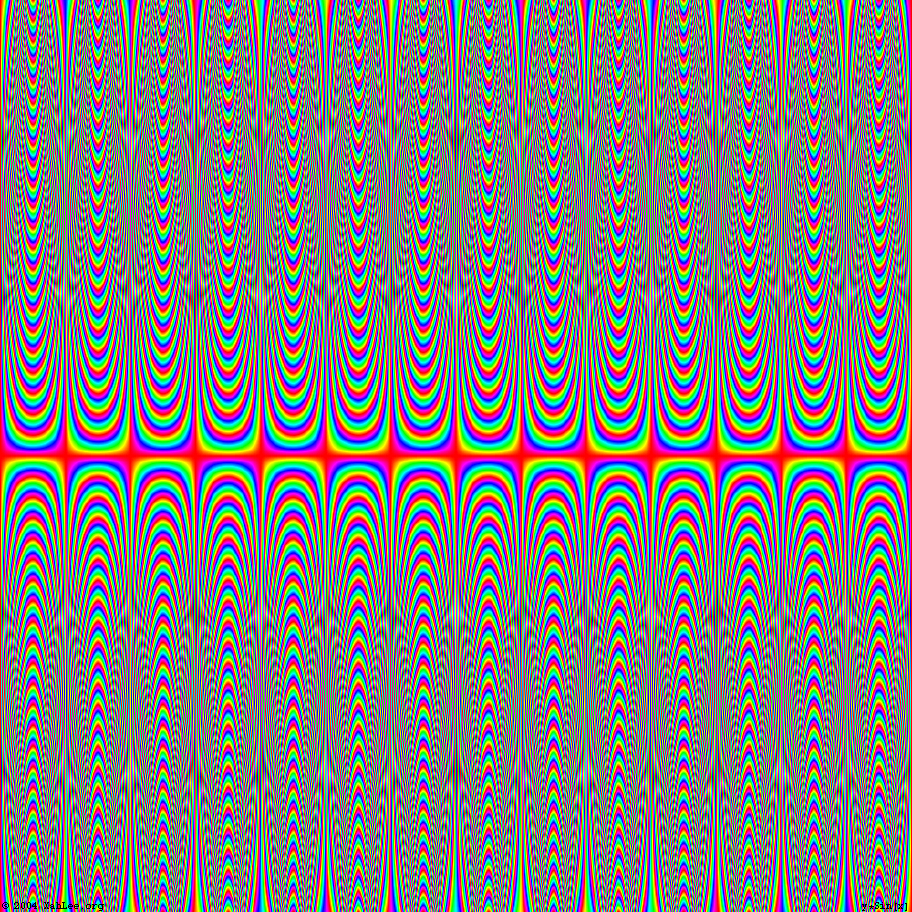y Sin[x](Csc[x] Sin[Sin[y] Tan[x]])/x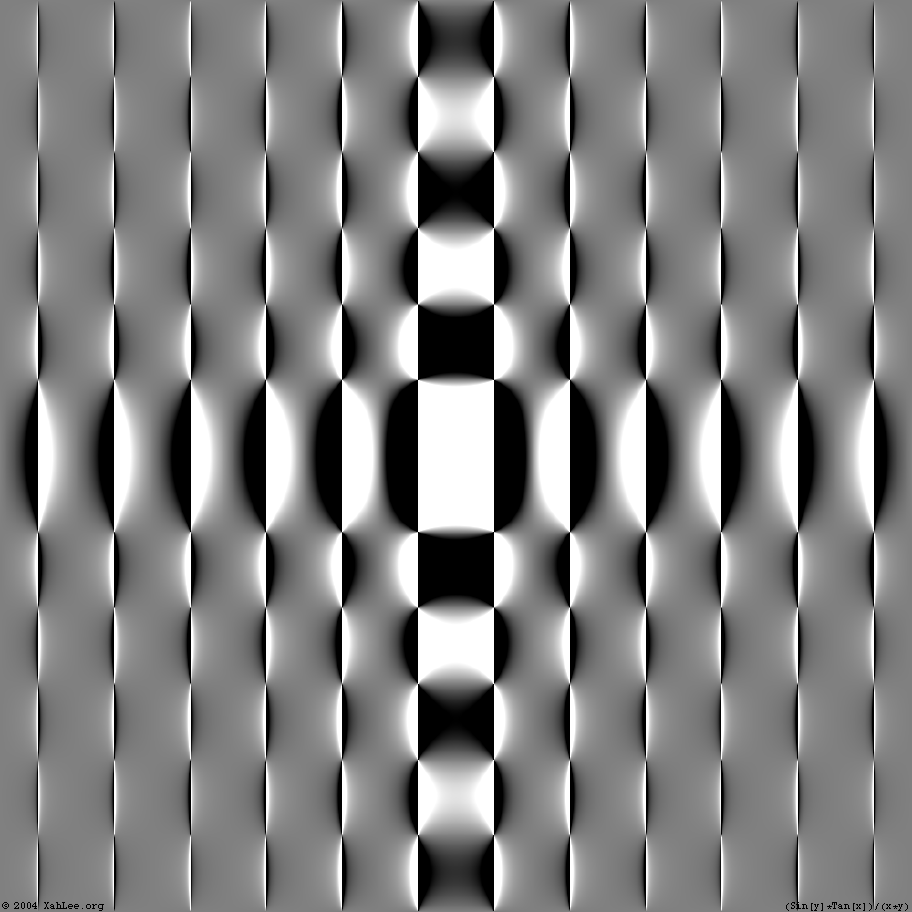(Sin[x] Tan[y])/(x y)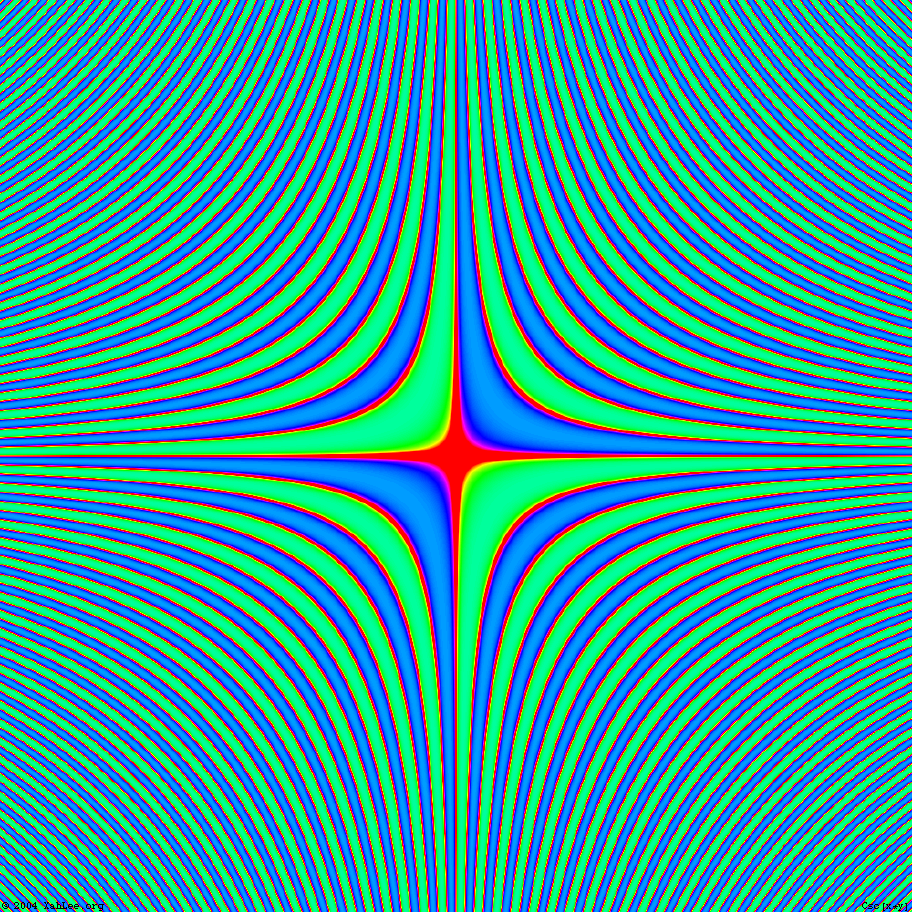Csc[x y]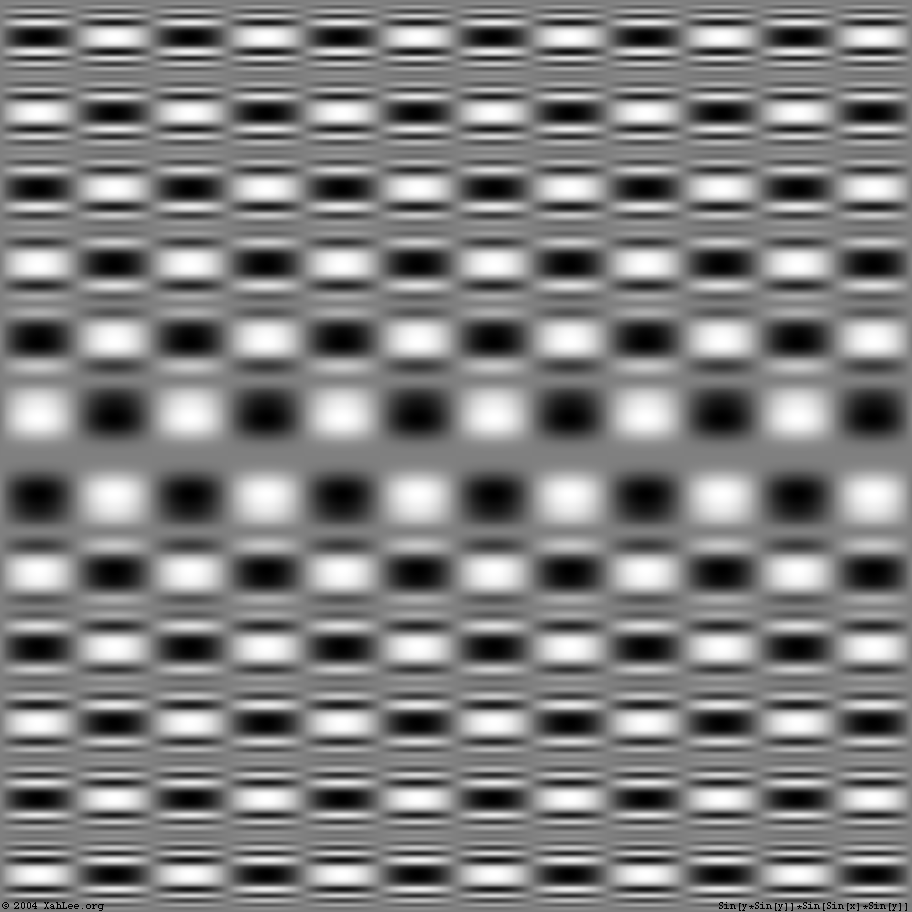Sin[y Sin[y]] Sin[Sin[x] Sin[y]]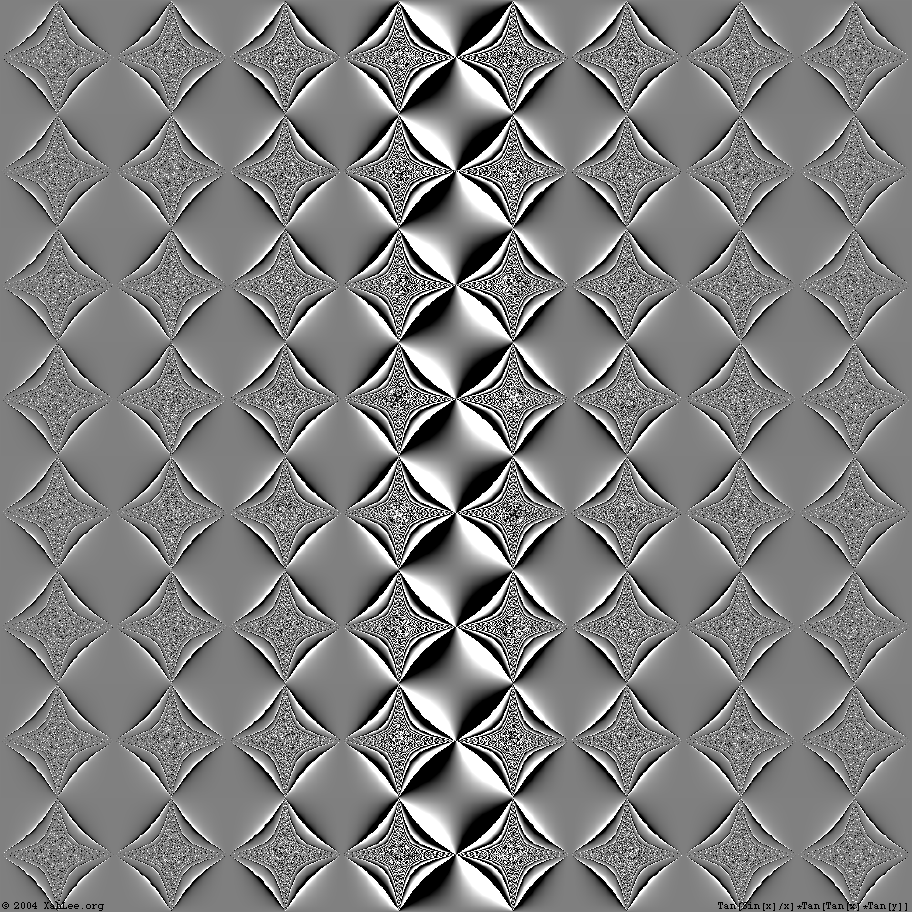Tan[ Sin[x]/x] Tan[ Tan[x] Tan[y]]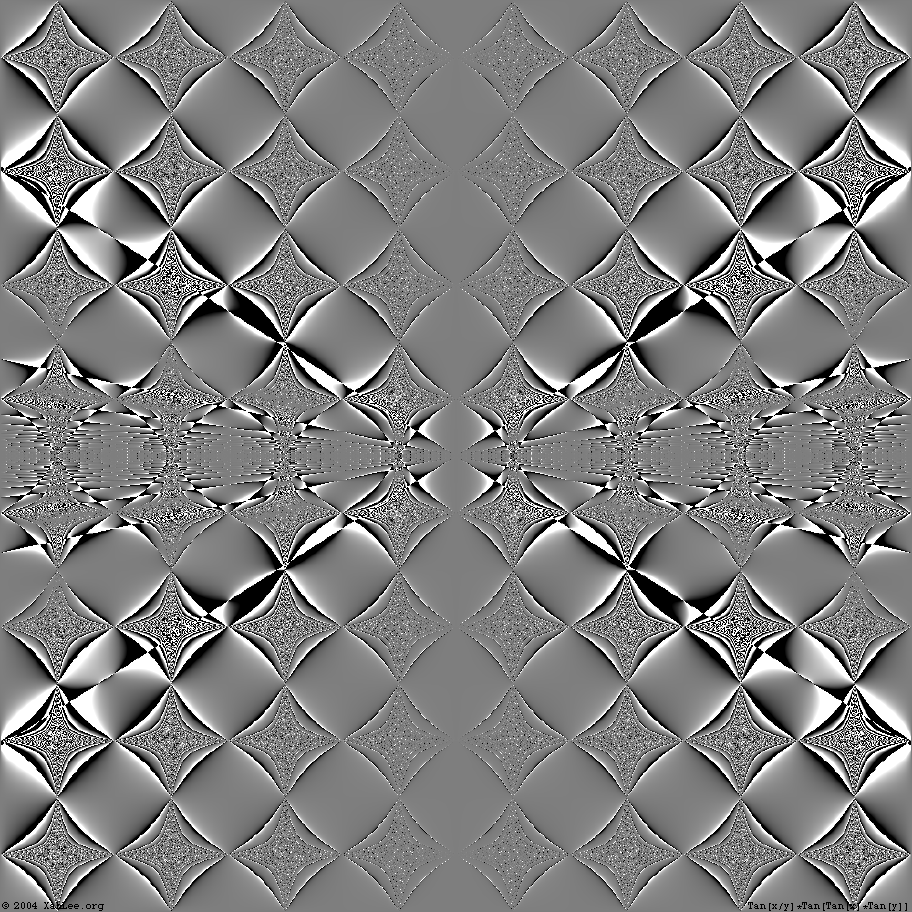Tan[ x/y] Tan[ Tan[x] Tan[y]]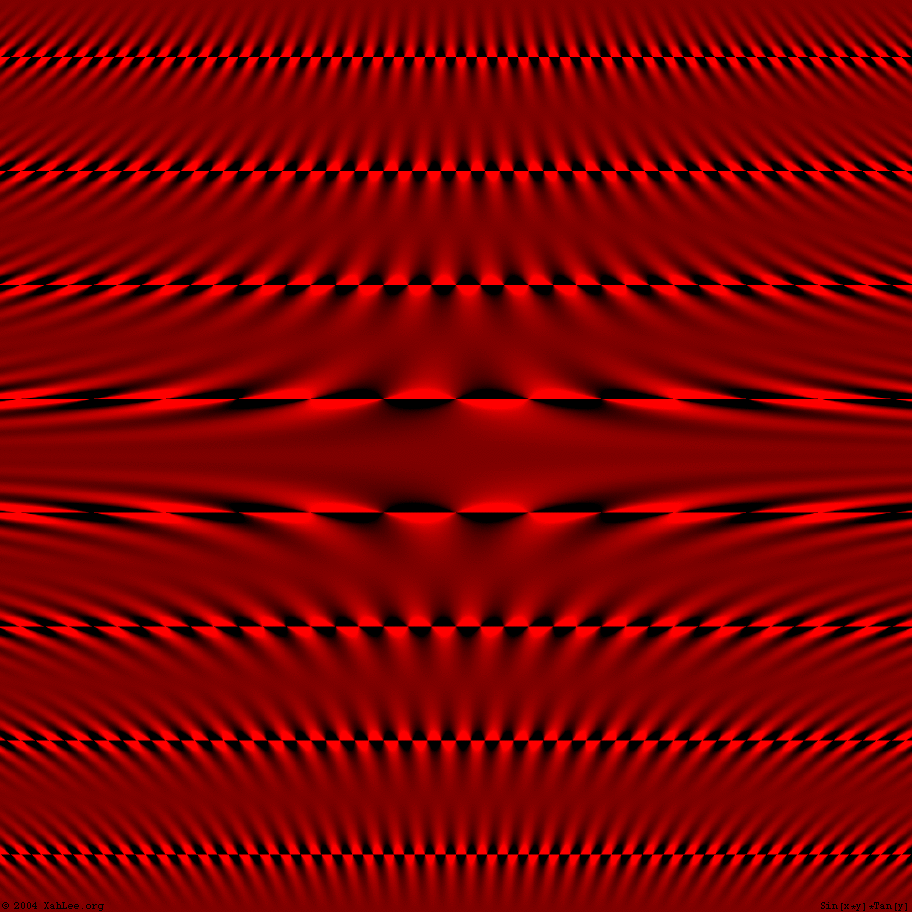Sin[x y] Tan[ y ]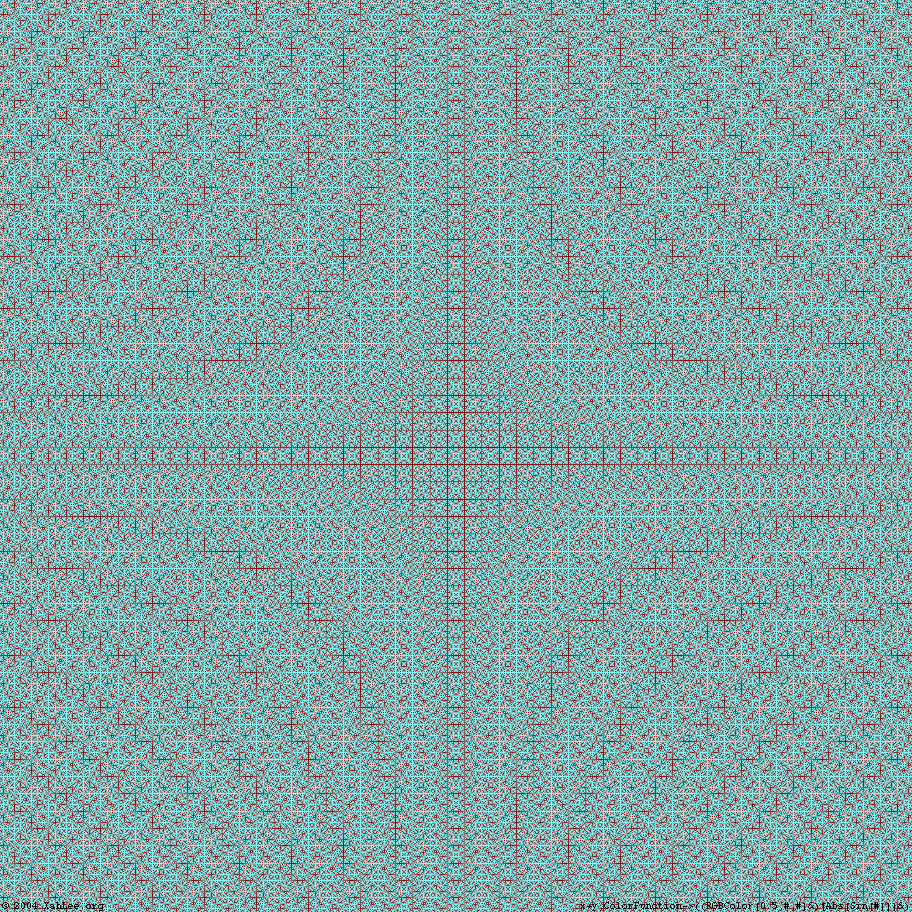x y, color by RGBColor[0.5, Abs[Sin[#]], Abs[Sin[#]]]&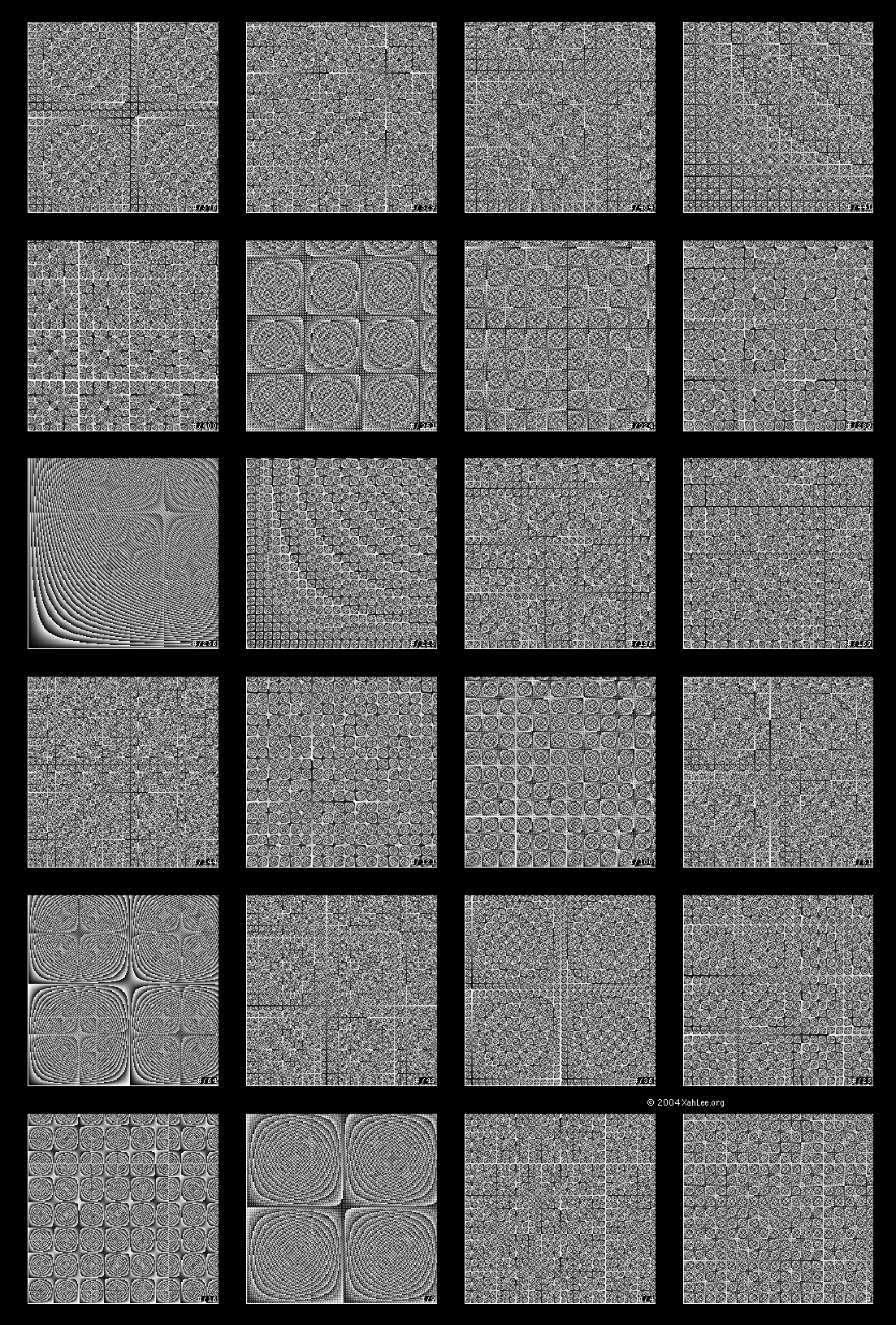morie

## Mathematica Code

The following is Mathematica code that generates all possible equations of one term. (tweak the funList and nesting level to define what “all possible” means. if nesting level is 2, it takes about 20 minutes and returns a list of some 2876 terms on a 2002 personal computer.

```<< DiscreteMath`Combinatorica`
funList = {Sin, Tan, Power[#, -1] &};
Nest[Module[{li = #},
(Union[#, SameTest -> (Module[{arg1 = #1, arg2 = #2},
If[(*both expression contains both x and y*)
And @@ (((((StringMatchQ[#, "*x*"] &&
StringMatchQ[#, "*y*"]) &)@
ToString@#) &) /@ {arg1, arg2})
, SameQ[arg1, arg2 /. {x -> y, y -> x}],
SameQ[arg1, arg2]]
] &)] &)@
Union@Flatten@(Table[(Times @@ # &) /@ KSubsets[#, i], {i, 1,
2}] &)@Flatten@{li, Outer[#1@#2 &, funList, li]}
] &, {x, y}, 1];
Select[%, (StringMatchQ[ToString@#, "*x*"] &&
StringMatchQ[ToString@#, "*y*"]) &]
```

Here are the first few items of the result, with nesting level at 2:

```{1/(x^2*y^2), 1/(x*y^2), x/y^2, 1/(x*y), x/y, x^2/y, x*y, x^2*y,
x^2*y^2, Cos[x]/y^2, Cos[x]/y, Cos[x]/(x*y), (x*Cos[x])/y, y*Cos[x],
(y*Cos[x])/x, x*y*Cos[x], y^2*Cos[x], Cos[x]*Cos[y], Cot[x]/y^2,
Cot[x]/(x*y^2)}
```

The problem is to enumerate all possible single-term expressions of 2 variables x and y, using all the trig functions, multiplication and its inverse, integer power and its inverse (roots), and nesting.

If you have a question, put \$5 at patreon and message me.